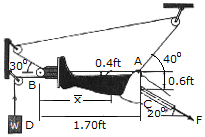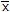# Engineering Mechanics - Equilibrium of a Rigid Body - Discussion

### Discussion :: Equilibrium of a Rigid Body - General Questions (Q.No.9)

9.A Russell's traction is used for immobilizing femoral fractures C. If the lower leg has a weight of 8 lb, determine the weight W that must be suspended at D in order for the leg to be held in the position shown. Also, what is the tension force F in the femur and the distancewhich locates the center of gravity G of the lower leg? Neglect the size of the pulley at B.

 [A]. x = 1.44 ft, w = 10.8 lb, F = 12.61 lb [B]. x = 1.33 ft, w = 15.76 lb, F = 20.0 lb [C]. x = 1.56 ft, w = 9.75 lb, F = 12.69 lb [D]. x = 0.869 ft, w = 6.44 lb, F = 5.03 lb

Explanation:

No answer description available for this question.